Ex 11.1

Chapter 11 Class 10 Constructions [Deleted]
Serial order wise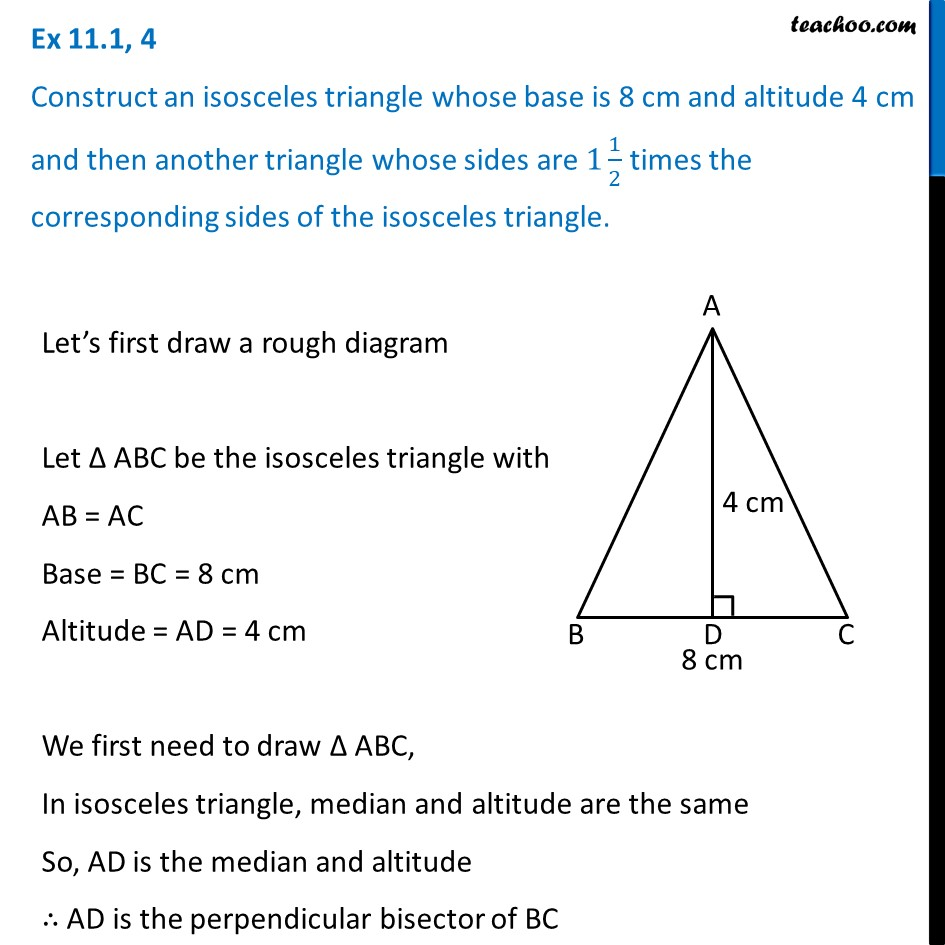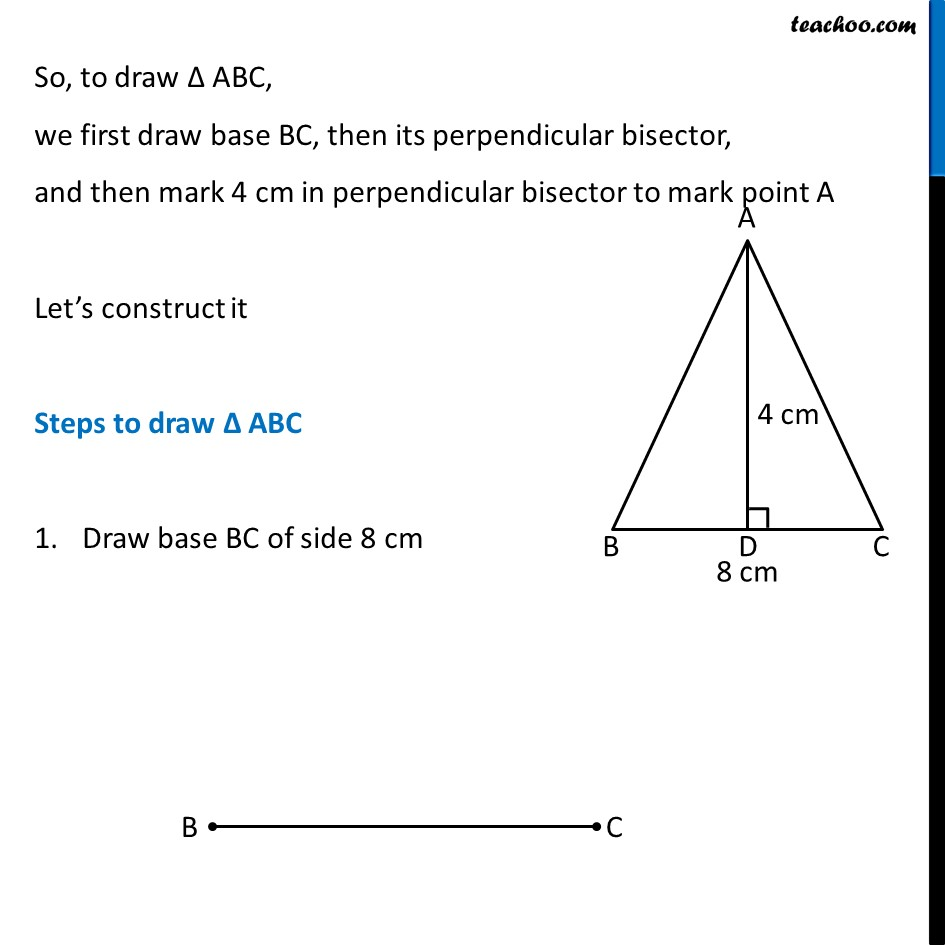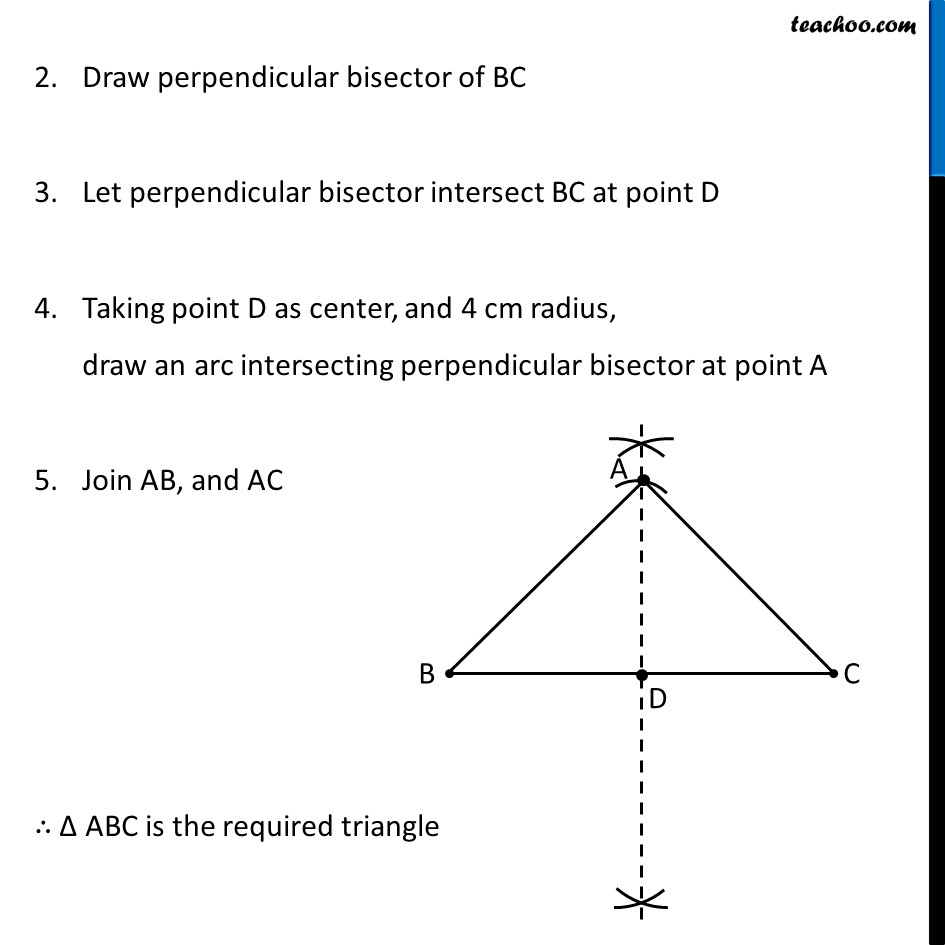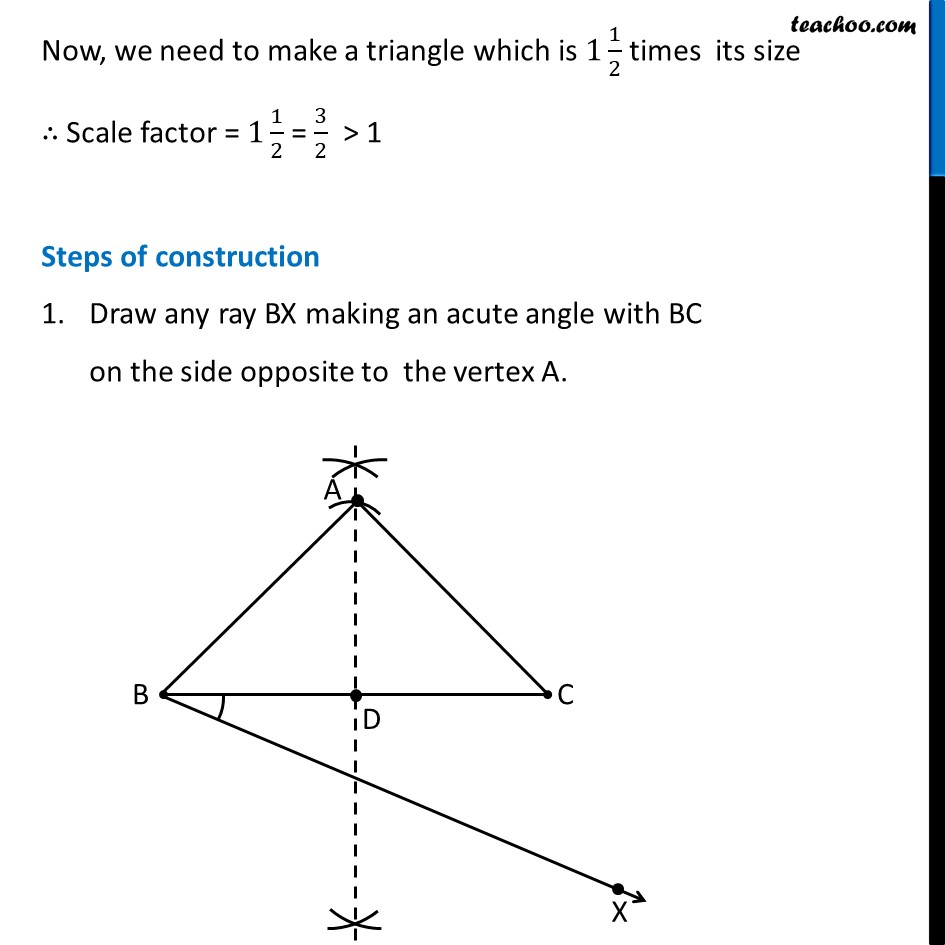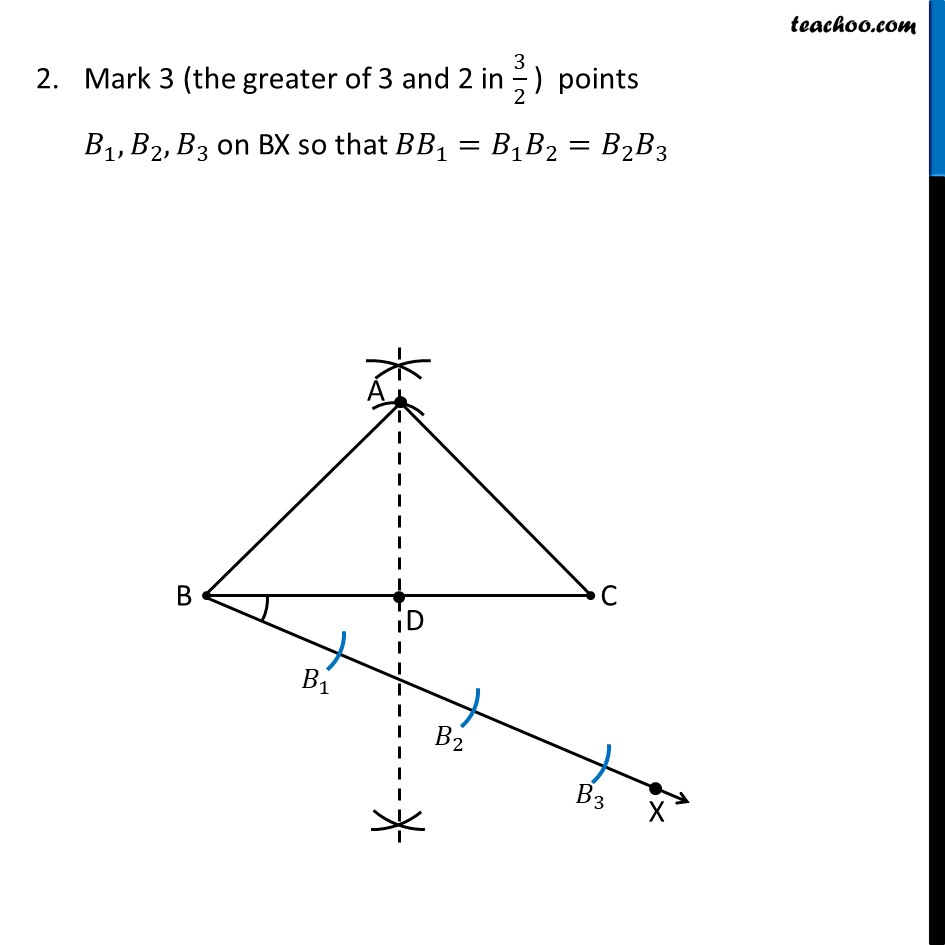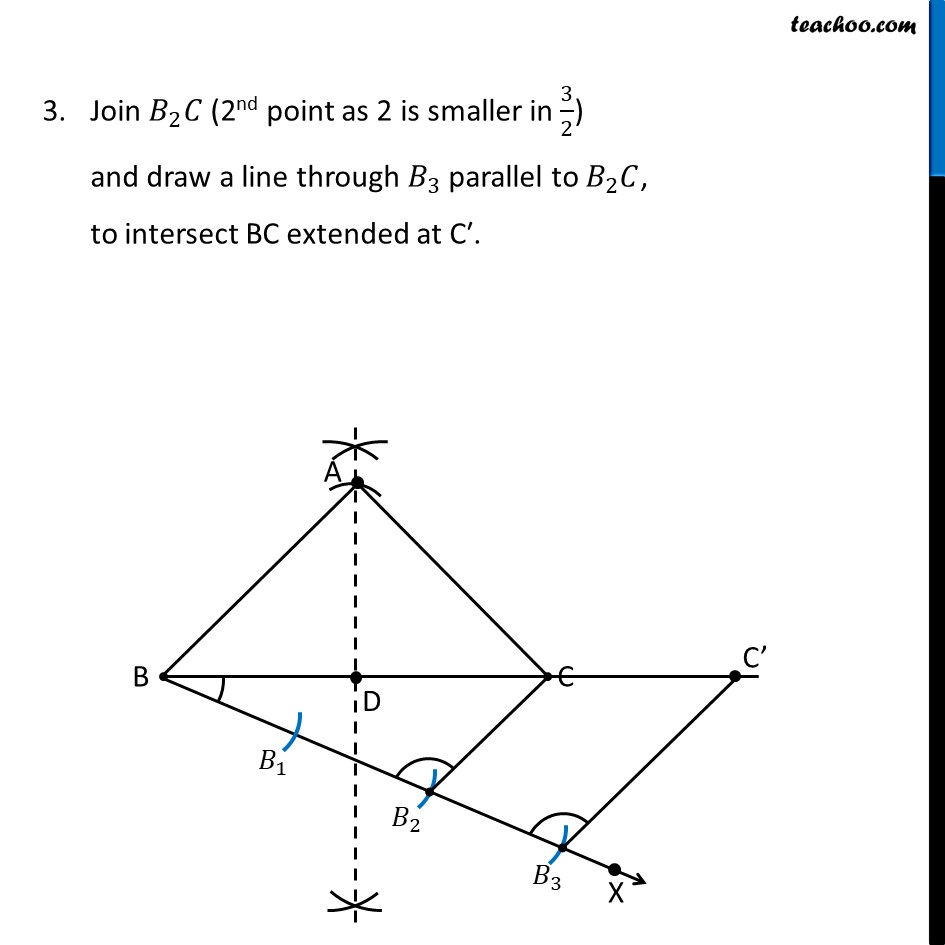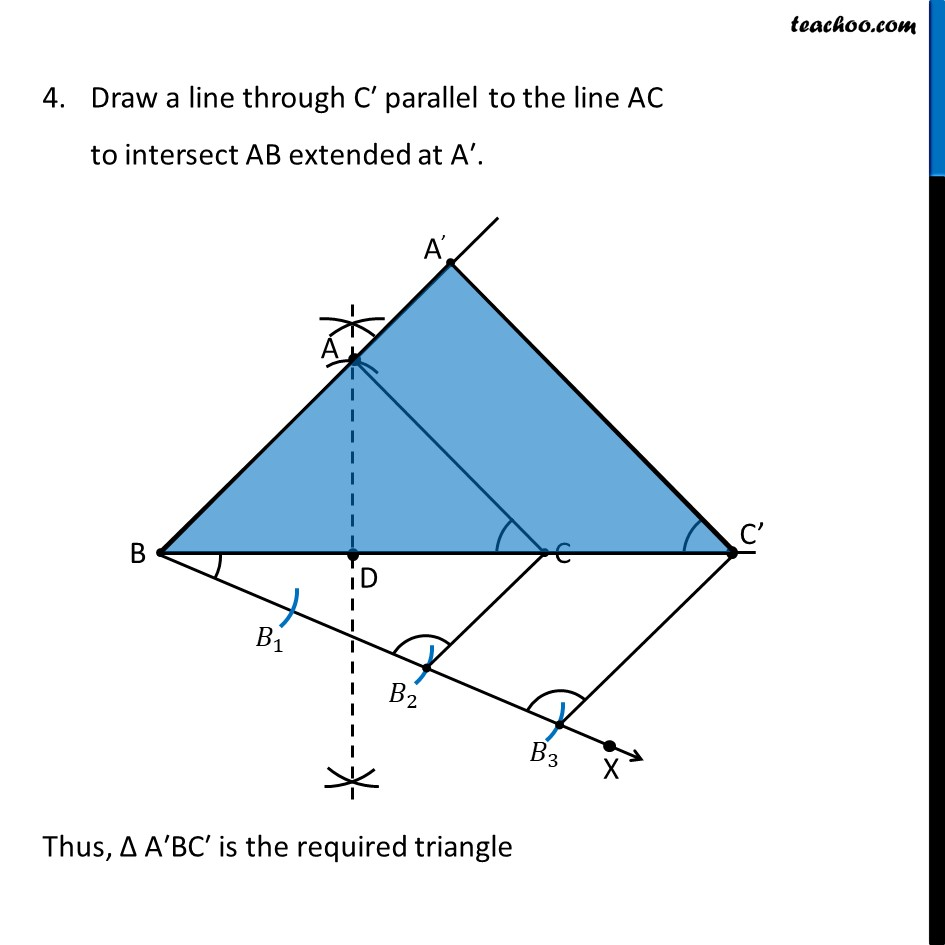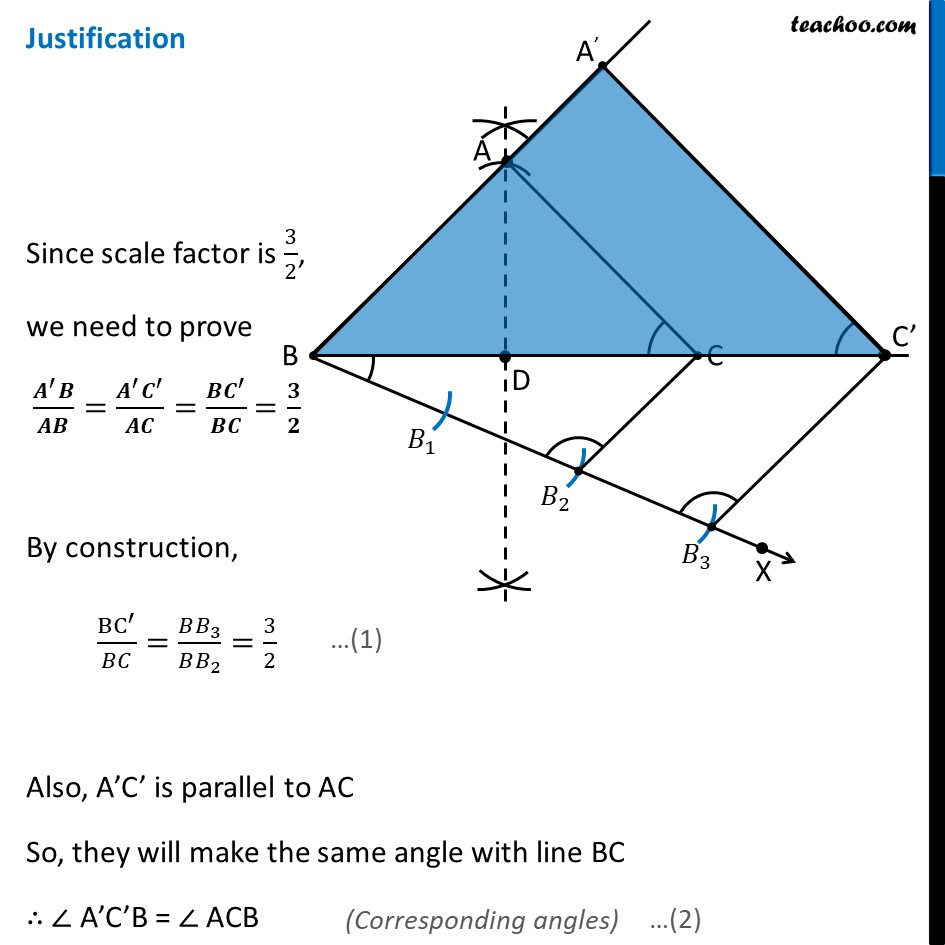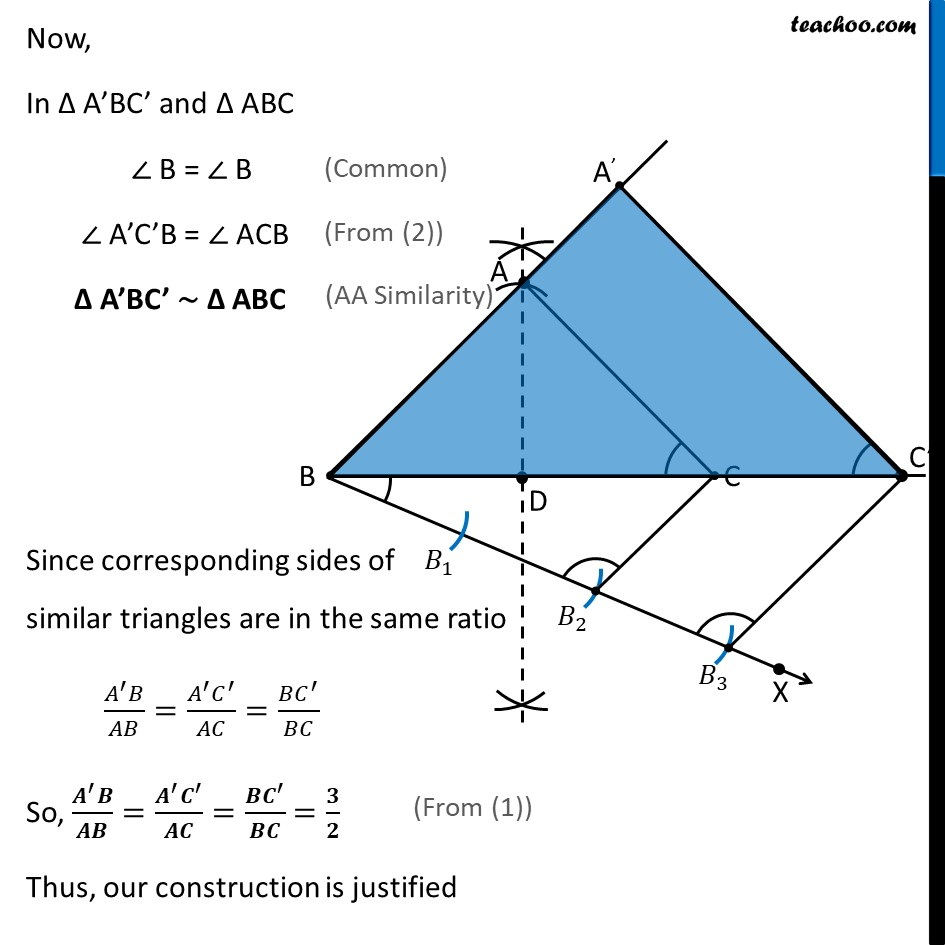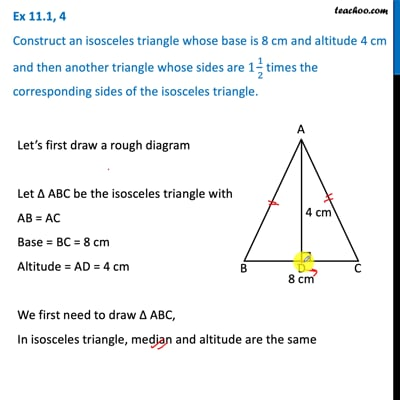This video is only available for Teachoo black users

Maths Crash Course - Live lectures + all videos + Real time Doubt solving!

### Transcript

Ex 11.1, 4 Construct an isosceles triangle whose base is 8 cm and altitude 4 cm and then another triangle whose sides are 1 1/2 times the corresponding sides of the isosceles triangle. Let’s first draw a rough diagram Let Δ ABC be the isosceles triangle with AB = AC Base = BC = 8 cm Altitude = AD = 4 cm We first need to draw Δ ABC, In isosceles triangle, median and altitude are the same So, AD is the median and altitude ∴ AD is the perpendicular bisector of BC So, to draw Δ ABC, we first draw base BC, then its perpendicular bisector, and then mark 4 cm in perpendicular bisector to mark point A Let’s construct it Steps to draw Δ ABC Draw base BC of side 8 cm Draw perpendicular bisector of BC Let perpendicular bisector intersect BC at point D Taking point D as center, and 4 cm radius, draw an arc intersecting perpendicular bisector at point A Join AB, and AC ∴ Δ ABC is the required triangle Now, we need to make a triangle which is 1 1/2 times its size ∴ Scale factor = 1 1/2 = 3/2 > 1 Steps of construction Draw any ray BX making an acute angle with BC on the side opposite to the vertex A. Mark 3 (the greater of 3 and 2 in 3/2 ) points 𝐵_1, 𝐵_2, 𝐵_3 on BX so that 〖𝐵𝐵〗_1=𝐵_1 𝐵_2=𝐵_2 𝐵_3 Join 𝐵_2 𝐶 (2nd point as 2 is smaller in 3/2) and draw a line through 𝐵_3 parallel to 𝐵_2 𝐶, to intersect BC extended at C′. Draw a line through C′ parallel to the line AC to intersect AB extended at A′. Thus, Δ A′BC′ is the required triangle Justification Since scale factor is 3/2, we need to prove (𝑨^′ 𝑩)/𝑨𝑩=(𝑨^′ 𝑪^′)/𝑨𝑪=(𝑩𝑪^′)/𝑩𝑪 =𝟑/𝟐 By construction, BC^′/𝐵𝐶=(𝐵𝐵_3)/(𝐵𝐵_2 )=3/2 Also, A’C’ is parallel to AC So, they will make the same angle with line BC ∴ ∠ A’C’B = ∠ ACB Now, In Δ A’BC’ and Δ ABC ∠ B = ∠ B ∠ A’C’B = ∠ ACB Δ A’BC’ ∼ Δ ABC Since corresponding sides of similar triangles are in the same ratio (𝐴^′ 𝐵)/𝐴𝐵=(𝐴^′ 𝐶^′)/𝐴𝐶=(𝐵𝐶^′)/𝐵𝐶 So, (𝑨^′ 𝑩)/𝑨𝑩=(𝑨^′ 𝑪^′)/𝑨𝑪=(𝑩𝑪^′)/𝑩𝑪 =𝟑/𝟐 Thus, our construction is justified